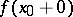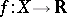# Discontinuity point

(diff) ← Older revision | Latest revision (diff) | Newer revision → (diff)
Jump to: navigation, search

A point in the domain of definitionof a function, whereandare topological spaces, at which this function is not continuous. Sometimes points that, although not belonging to the domain of definition of the function, do have certain deleted neighbourhoods belonging to this domain are also considered to be points of discontinuity, if the function does not have finite limits (see below) at this point.

Among the points of discontinuity of a function, defined on deleted neighbourhoods of points on the real axis, one distinguishes points of the first and second kind. If a pointis a point of discontinuity of a functionthat is defined in a certain neighbourhood of this point, except perhaps at the point itself, and if there exist finite limits from the leftand from the rightfor(in a deleted neighbourhood of), then this point is called a point of discontinuity of the first kind and the numberis called the jump ofat. If moreover this jump is zero, then one says thatis a removable discontinuity point. If the discontinuity point is not of the first kind, then it is a discontinuity point of the second kind.

#### Comments

The set of discontinuity points of a function,a topological space, is always a countable union of closed sets (and a countable union of closed sets is the set of discontinuity points of a real-valued function ifis Hausdorff, cf. Hausdorff space). This fact is related to the Baire theorem. Cf. also Baire classes and [a1].

A point of discontinuity of the first (respectively, second) kind is also called a jump point (respectively, an oscillatory discontinuity). Functions defined on an interval ofand free of oscillatory discontinuities are intensively used in the theory of stochastic processes (cf. Stochastic process), where they are often called, after the French, làglàd functions, (respectively, càglàd functions) if they are right (respectively, left) continuous.

How to Cite This Entry:
Discontinuity point. Encyclopedia of Mathematics. URL: http://encyclopediaofmath.org/index.php?title=Discontinuity_point&oldid=12112
This article was adapted from an original article by L.D. Kudryavtsev (originator), which appeared in Encyclopedia of Mathematics - ISBN 1402006098. See original article